# MRI Graph Analysis BUD

GUIMRIGraphAnalysisBUD is a graphical user interface that allows the user to perform a brain graph analysis of MRI data using binary undirected graphs at a fixed density of connections (BUD = Binary Undirected Density). The user can calculate group measures, compare them with random graphs, and compare the measures of two groups by permutation test. Significance intervals and single/double-tailed p-values are provided (the p-values can be corrected for false discovery rate (FDR) in the case of nodal measures). Global and nodal measures are displayed separately; for nodal measures the user has the option to visualize the results on a brain surface. The graph analysis can be saved in a file *.mga for future use within BRAPH; it can also be exported in xml format for use within other programs.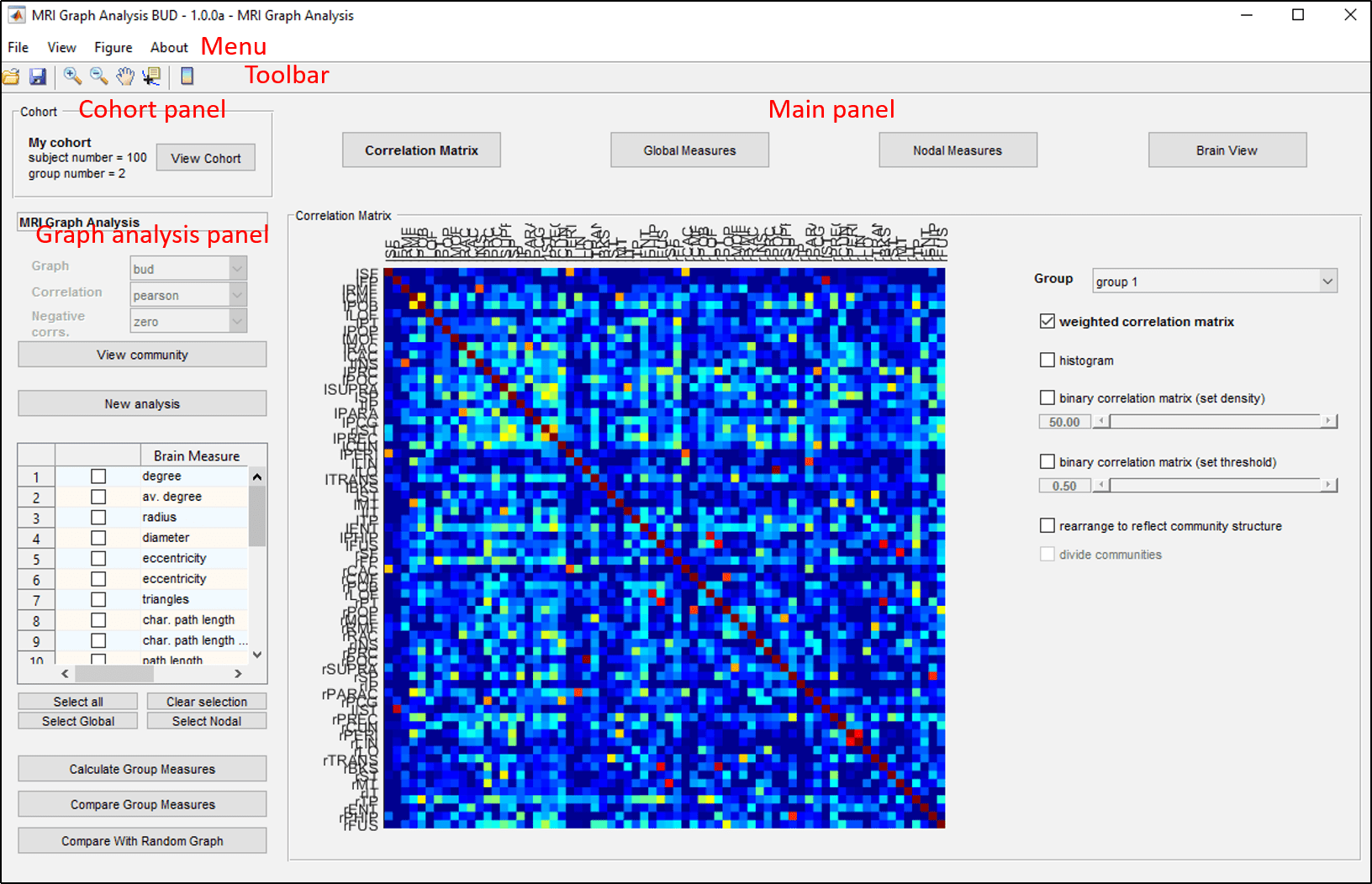Figure 1: Snapshot of GUIMRIGraphAnalysisBUD. On the top, there are the menu and the toolbar; below, there are the cohort panel and the graph analysis panel (on the left), and the main panel (on the right).

The layout of GUIMRIGraphAnalysisBUD is shown in figure 1. It is composed of five main work areas:

• Menu permits one to access the basic functionalities of GUIMRIGraphAnalysisBUD, including loading and saving an MRI graph analysis.
• Cohort panel permits one to view the MRI cohort properties.
• Graph analysis panel permits one to choose which measures to calculate or compare, view the community structure, and, if needed, start a new graph analysis.
• Main panel allows one to view the connectivity matrix as well as the calculated global and nodal measures and comparisons.
Getting Started

As a first example of the use of GUIMRIGraphAnalysisBUD, we will proceed to calculate some global (average degree, clustering coefficient) and local (degree, triangles) measures, and to compare the results for two groups. Then, we will visualize these results and the graph on the brain surface. Finally, we will save the graph analysis in a *.mga file.

1. In the graph analysis view, select from the list of measures the average degree, diameter, degree, and triangles measures.
To quickly select more than one measure, use the buttons below the measure list:

• Select all selects all the measures.
• Clear selection clears the current selection.
• Select Global selects all global measures.
• Clear Nodal selects all nodal measures.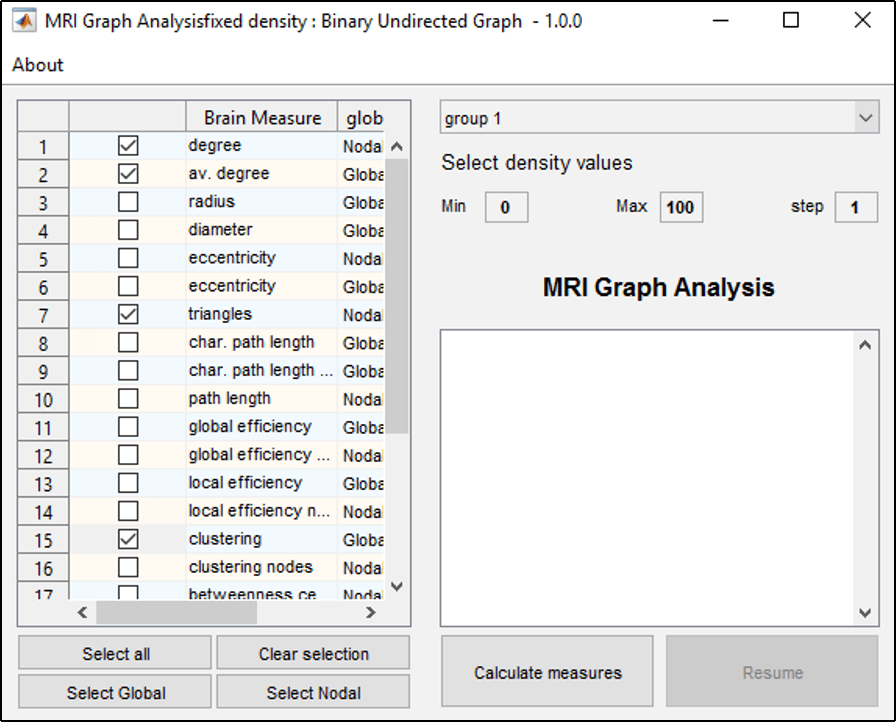Figure 2: Interface to calculate group measures.

2. Push Calculate Group Measures in the graph analysis panel (figure 1) to calculate the selected measures. This opens a new interface, shown in figure 2. On the left of the interface, there is a list with the selected measures to be calculated. On the right, there are a popup menu to select the group for which the measures will be calculated and a series of fields to enter the density values (including the minimum density, the maximum density, and the density step). The calculation is started by pushing Calculate measures. When the calculation is in progress, the status of the button changes to stop and pushing it stops the calculation; the calculation can then be resumed by pressing Resume.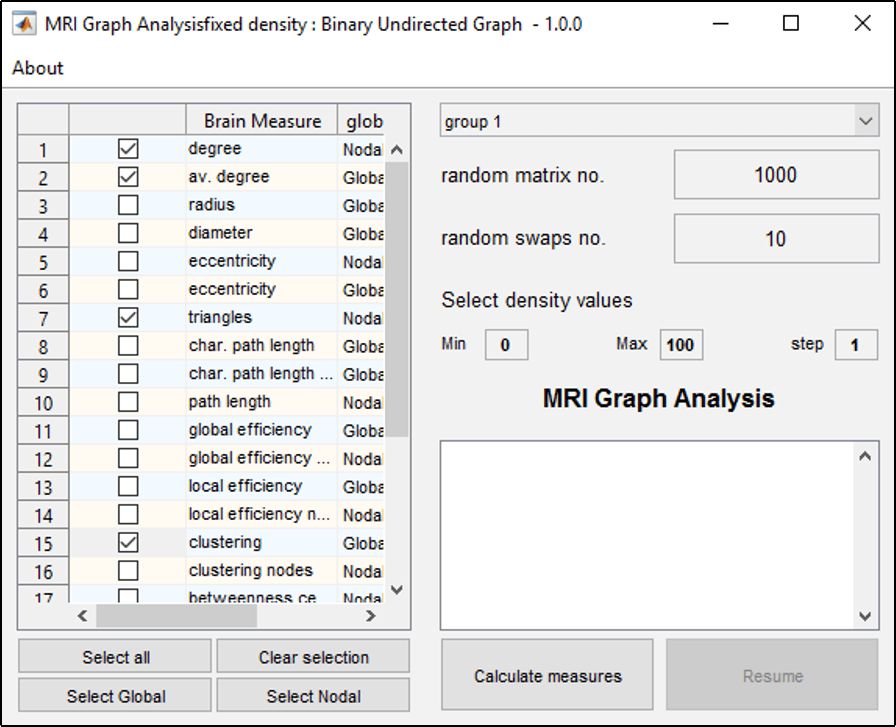Figure 3: Interface to calculate group measures normalized by comparison with random graphs.

3. Push Compare with Random Graph in the graph analysis panel (figure 1)  to calculate measures that are normalized by the results obtained from random graphs. This opens the interface shown in figure 3. This interface is analogous to that to calculate group measures, which we have seen in the previous step, but two new parameters can be inputted:
• random matrix no. sets how many random graphs are used in the comparison.
• random swaps no. sets how many times each edge is rewired to randomize the original graph.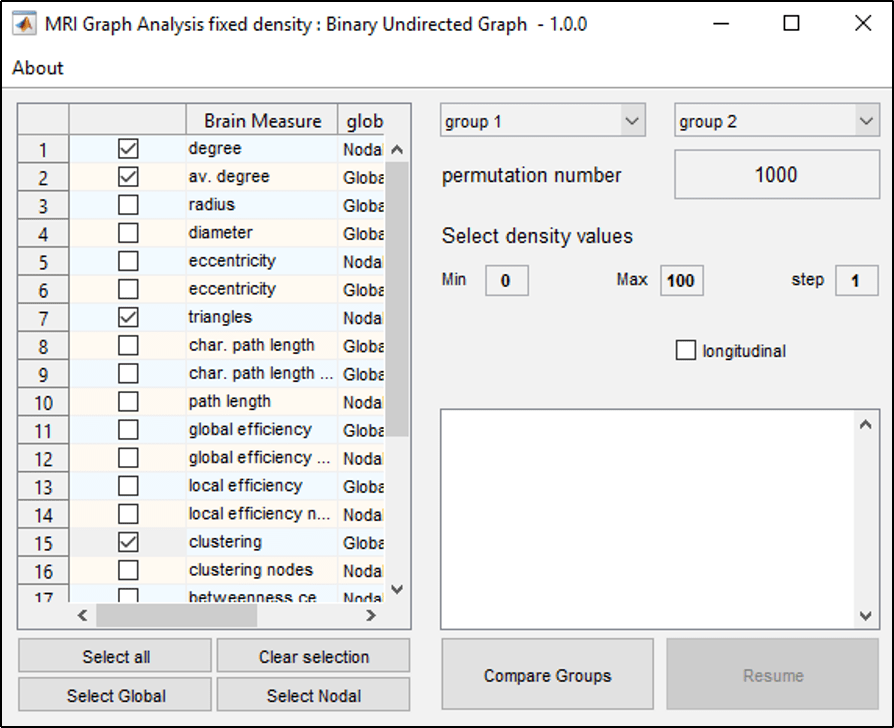Figure 4: Interface to compare the measures of two groups.

4. Push Compare Group Measures in the graph analysis panel (figure 1) to compare the measures between two groups. This opens the interface shown in figure 4. This interface is analogous to that to calculate group measures, but with two new parameters:
• permutation number sets how many permutations are performed in the permutation test.
• longitudinal sets whether the comparison is done for longitudinal data.Figure 5: Global measures tab. Here the average degree is shown as a function of the density.

5. Push Global Measures in the main panel to visualize the results for the global measures (figure 5).
If the measure checkbox is checked, group measures are displayed (the measure and the group are selected using the popup menus at the bottom). If the comparison checkbox is checked, comparisons between two groups are displayed (the measure and the groups are selected using the popup menus at the bottom). If the random comparison checkbox is checked, measures normalized by comparison with random graphs are displayed (the measure and the group are selected using the popup menus at the bottom).The main panel now shows two parts:

1. A table view on the left shows the numerical information about the selected measure. Among other data, this includes the value of the measure, the group for which it was calculated, and the density and threshold of the graph on which it was calculated. If it is a comparison between groups or with random graphs, the difference between the values and the single/double-tailed p-values are also displayed. A series of options are available below the table view:
• Select all selects all the measures.
• Clear selection clears the current selection.
• Remove removes the selected measure.
2. A graph view plots a graph of the selected measure as a function of density. By pressing Ctrl+F the graph can be exported as a figure that can be customized using the standard MatLab plotting tools; then, the figure can be exported in several standard graphic formats. Below the graph, several options are available to customize the plot: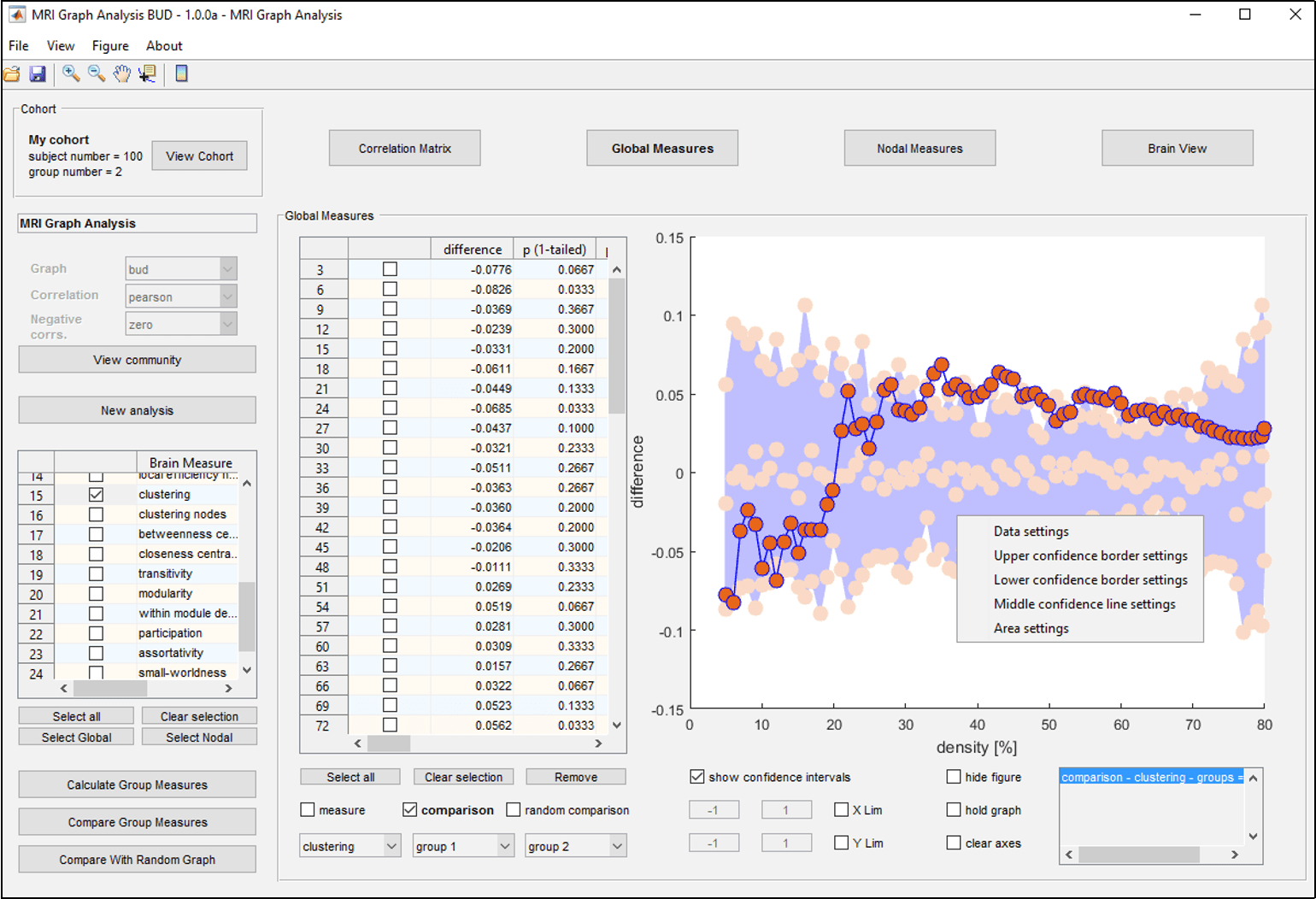Figure 6: Confidence intervals when comparing two groups.

• show confidence intervals shows the 95% confidence intervals as shown in figure 6. This option is only available for comparisons between groups and with random graphs. As can be seen in the figure 6 by right-clicking on the confidence intervals, a popup menu appears which allows the user to change the properties of the data values, lower, middle, and higher borders of the confidence interval as well as of the area plot properties.
• When X Lim is checked, the x-limits of the axes can be entered from the two neighboring edit boxes. If not checked, the limits are automatically set.
• When Y Lim is checked, the y-limits of the axes can be entered from the two neighboring edit boxes. If not checked, the limits are automatically set.
• When hide figure is checked, the graph is hidden and the table is extended.
• When hold graph is checked, more than one data set can be plotted on the same graph. The plotted measures are listed below the graph on the right.
• clear axes erases all the data plotted on the graph.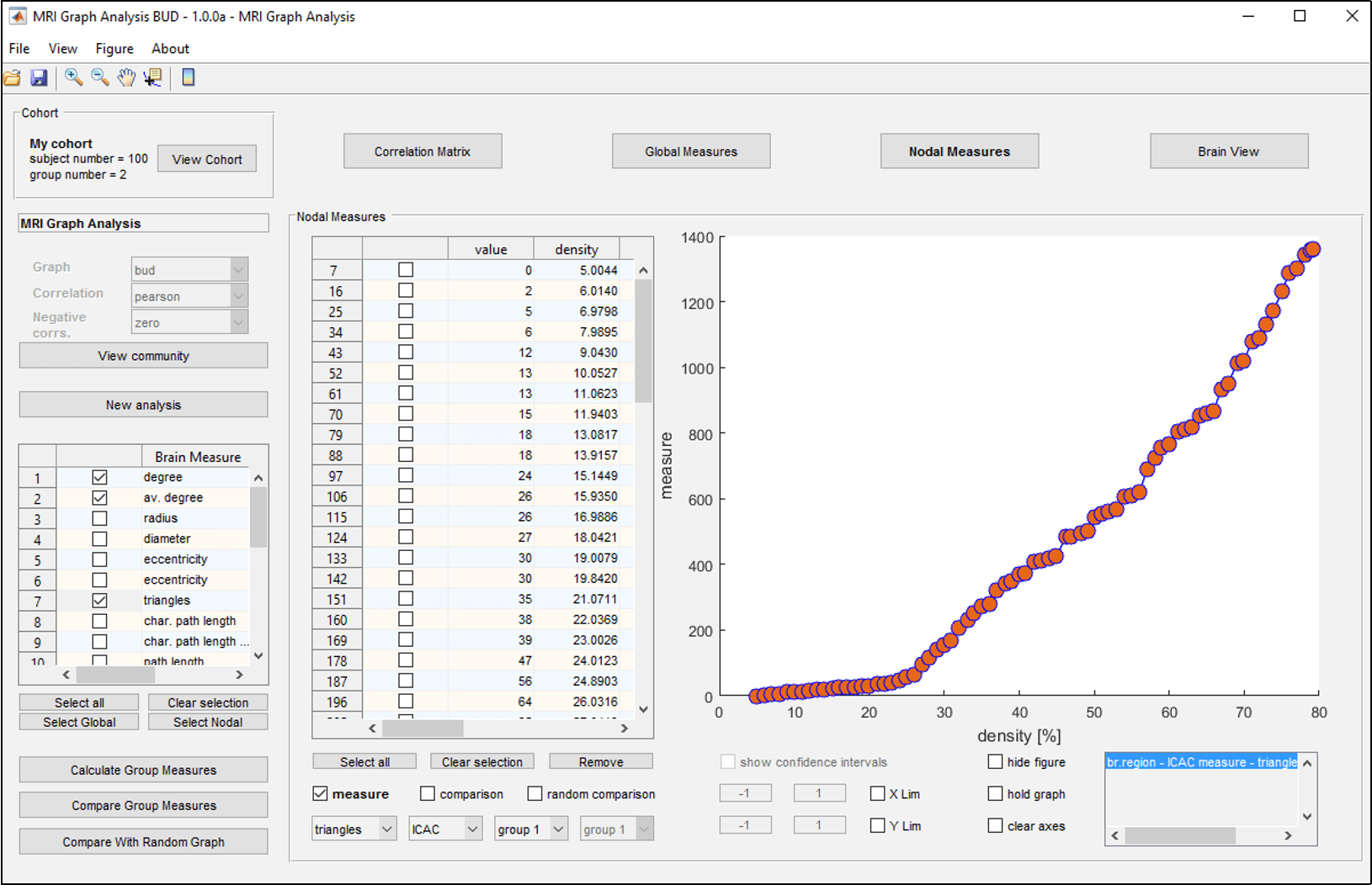Figure 7: Nodal measures tab.

6. Push Nodal Measures in the main panel to visualize the results for nodal measures (figure 7). This interface is very similar to that explained above for the global measures. The main difference is that now it is possible to select also the brain region.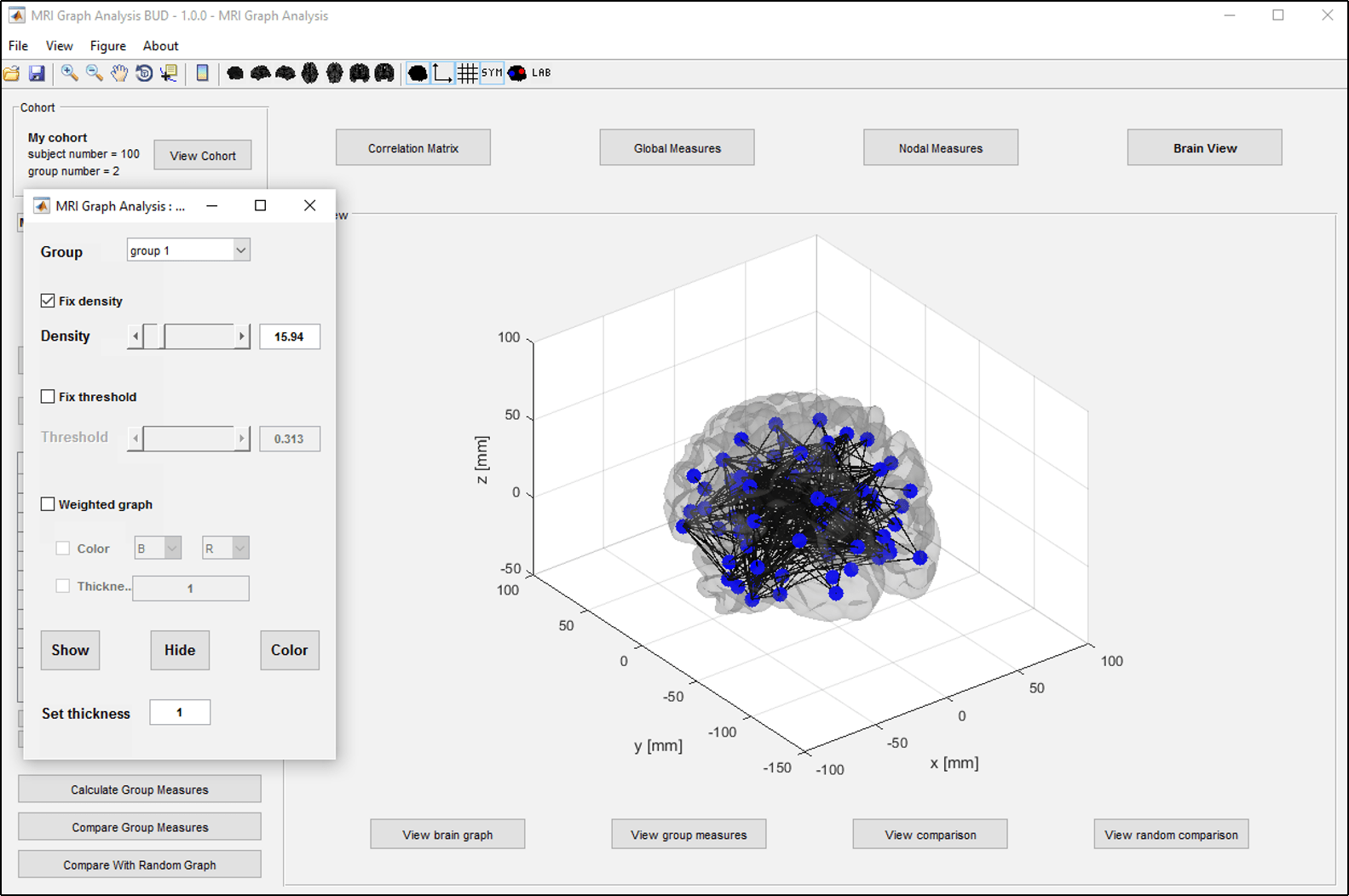Figure 8: Visualization of the brain graph on the brain surface.

7. Push Brain View in the main panel to visualize the nodal measures on a brain surface. By pushing the buttons at the bottom of this panel, the user can visualize the brain graph, the group measures, the comparisons between two groups, and the comparisons with random graphs.
• View brain graph visualizes the brain graph, as shown in figure 8. The graph parameters that can be specified are:– fix density draws the binary brain graph with the selected density of connections. The density can be specified by entering the value in the corresponding field or by using the slider.
fix threshold draws the binary brain graph with all connections having larger weight than the given threshold. The threshold can be specified by entering the value in the corresponding field or by using the slider.
weighted plots all the connection of the weighted brain graph. The weight of the connections can be encoded by color and/or thickness (by checking the corresponding checkboxes).
Show shows the current graph on the brain surface.
Hide hides the current graph from the brain surface.
Color plots the current graph in the specified color.
Set thickness sets the thickness of the connections.Figure 9: Visualization of the nodal measures on the brain surface.

• View group measures visualizes the nodal measures calculated for a given group, as shown in figure 9. The group for which the measures are to be shown is selected from the popup menu in the top left. In the first list below it, the user can select the measure; the list on the left shows the densities for which the measure have been calculated.Figure 10: Visualization of the comparison between two groups on the brain surface.

• View comparison visualizes the comparison between two groups, as shown in figure 10. The two popup menus on the left specify the two groups that are compared and the lists below them show the measures and the densities for which they have been calculated. The other functionalities are analogous to the interface for viewing group measures with two new options:

fdr (1-tailed), if checked, corrects the p-values for single-tailed false discovery rate. Only brain regions with significant p-values are then shown on the brain surface.
fdr (2-tailed), if checked, corrects the p-values for double-tailed false discovery rate. Only brain regions with significant p-values are then shown on the brain surface.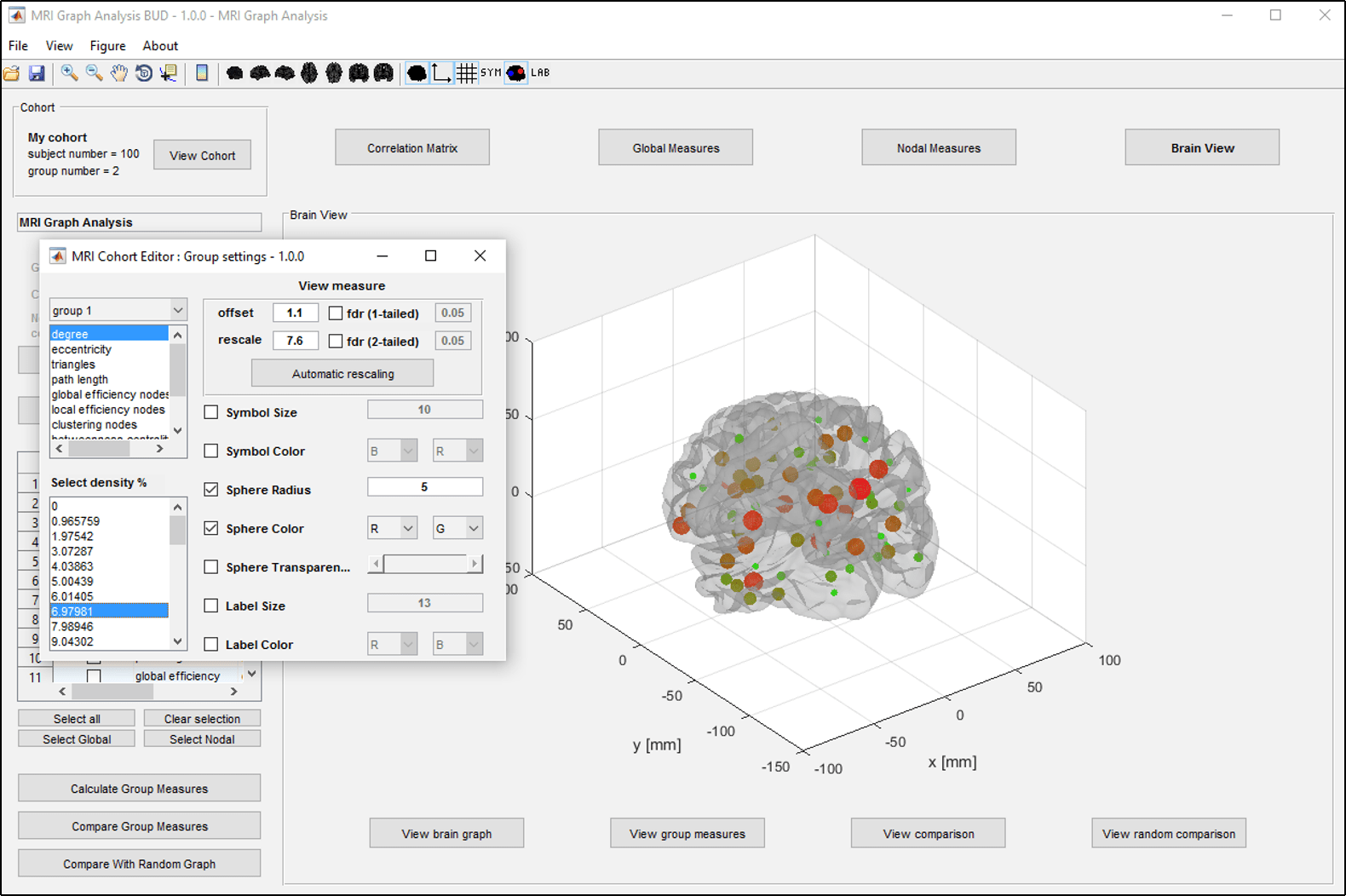Figure 11: Visualization of the measures normalized by random comparison on the brain surface.

• View random comparison visualizes the measures normalized by comparing them with random graphs, as shown in figure 11. All the functionalities of this interface are analogous to those of the interface to visualize a comparison between groups.
• Select File$\rightarrow$ Save to save the MRI graph analysis BUD as a *.mga file; alternatively you can also use the shortcut Ctrl+S or the Save icon on the toolbar.
• Select File$\rightarrow$ Open to open an MRI graph analysis BUD previously saved with GUIMRIGraphAnalysisBUD; alternatively you can also use the shortcut Ctrl+O or the Open icon on the toolbar.

Cohort panel

The cohort is already uploaded when GUIMRIGraphAnalysisBUD is launched. The cohort view shows the cohort’s properties including name, number of subjects, and groups. Moreover, all cohort’s properties can be viewed in the GUIMRICohort interface with restricted access by pushing View Cohort.

Graph analysis panel

The graph analysis panel shows the information relative to the graph analysis and gives the user the ability to calculate measures. The available features are:

• Graph analysis properties. These three popup menus show the properties of the graph analysis: graph type, correlation type, and how to deal with negative correlations. As all these properties were set in GUIMRIGraphAnalysis, here all the popup menus are disabled and the properties cannot be changed. If the user wishes to change any of the properties, a new graph analysis should be started.
• View community opens the interface to view the community structure that is set. Also the community structure cannot be changed.
• New analysis opens a new GUIMRIGraphAnalysis interface where a new graph analysis with different parameters can be launched.
• Measure list lists all measures available for calculation for binary graphs. The user can choose which measure to calculate by checking the checkboxes next to them.
• Calculate Group Measures opens an interface allowing the user to set parameters to calculate the selected measures.
• Compare Group Measures opens an interface allowing the user to set parameters to compare the selected measures between two groups.
• Compare With Random Graphs opens an interface allowing the user to set parameters to compare the selected measures with random graphs.

Main Panel

The main panel consists of a main table that displays different information about the graph analysis and the calculated measures. The console buttons are used to switch between the various types of information shown in the table. The following information can be displayed:

• Correlation Matrix visualizes the connectivity matrix based on the parameters set on the right. For more detailed information about how to visualize the connectivity matrix please refer to the section Main view of the chapter MRI Graph Analysis.
• Global Measures allows the user to visualize global measures.
• Nodal Measures allows the user to visualize nodal measures.
• Brain View allows the user to visualize the brain graph and the calculated nodal measures on a brain surface.

File provides various options for importing and saving an MRI graph analysis BUD:

• File$\to$ Open (Ctrl+O) opens a popup window to load an MRI graph analysis BUD saved in *.mga format.
• File$\to$ Close (Ctrl+C) closes the GUIMRIGraphAnalysisBUD.
• File$\to$ Save (Ctrl+S) saves the current MRI graph analysis BUD in *.mga format.
• File$\to$ Save as opens a popup window to save the current MRI graph analysis BUD in *.mga format possibly in a different file.
• File$\to$ Import (xml) imports an MRI graph analysis BUD from an xml file.
• File$\to$ Export (xml) exports the current MRI graph analysis BUD to an xml file.

View switches the main view to display various types of information:

• View$\to$ Correlation Matrix visualizes the connectivity matrix.
• View$\to$ Global Measures visualizes global measures.
• View$\to$ Nodal Measures visualizes nodal measures.
• View$\to$ Brain View visualizes the data on a brain surface.

Figure$\to$ Generate figure (Ctrl+F) generates a figure that can be customized using the standard MatLab plotting tools. The figure can then be exported in several standard graphic formats.

About$\to$ About provides information about the current version of GUIMRIGraphAnalysisBUD and BRAPH.

Toolbar

The toolbar provides different options to open and save the MRI graph analysis BUD and visualize the figures. It is shown in figure 12.Figure 12: MRIGraphAnalysisBUD toolbar.

Open and save commands

These commands allow the user to open and save an MRI graph analysis BUD in the *.mga format. These are equivalent to the open and save menu options in the File menu.opens a popup window to load an MRI graph analysis BUD saved in *.mga format.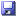saves the current MRI graph analysis BUD in *.mga format.

Visualization commands

These commands allow the user to control the visualization of the graphical representations.zooms in image.zooms out image.drags image.shows/hides data cursor.shows color scale.standard 3D view.sagittal left view.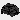sagittal right view.axial dorsal view.axial ventral view.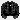coronal anterior view.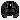coronal posterior view.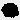switches brain surface on/off.switches axis on/off.switches grid on/off.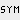switches brain region symbols on/off.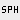switches brain regions spheres on/off.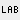switches brain region labels on/off.

Footnotes and references

1. ^ For more information about the rescaling and the filters needed to be applied to visualize the data, refer to the Main panel subsection in the chapter MRI Cohort.